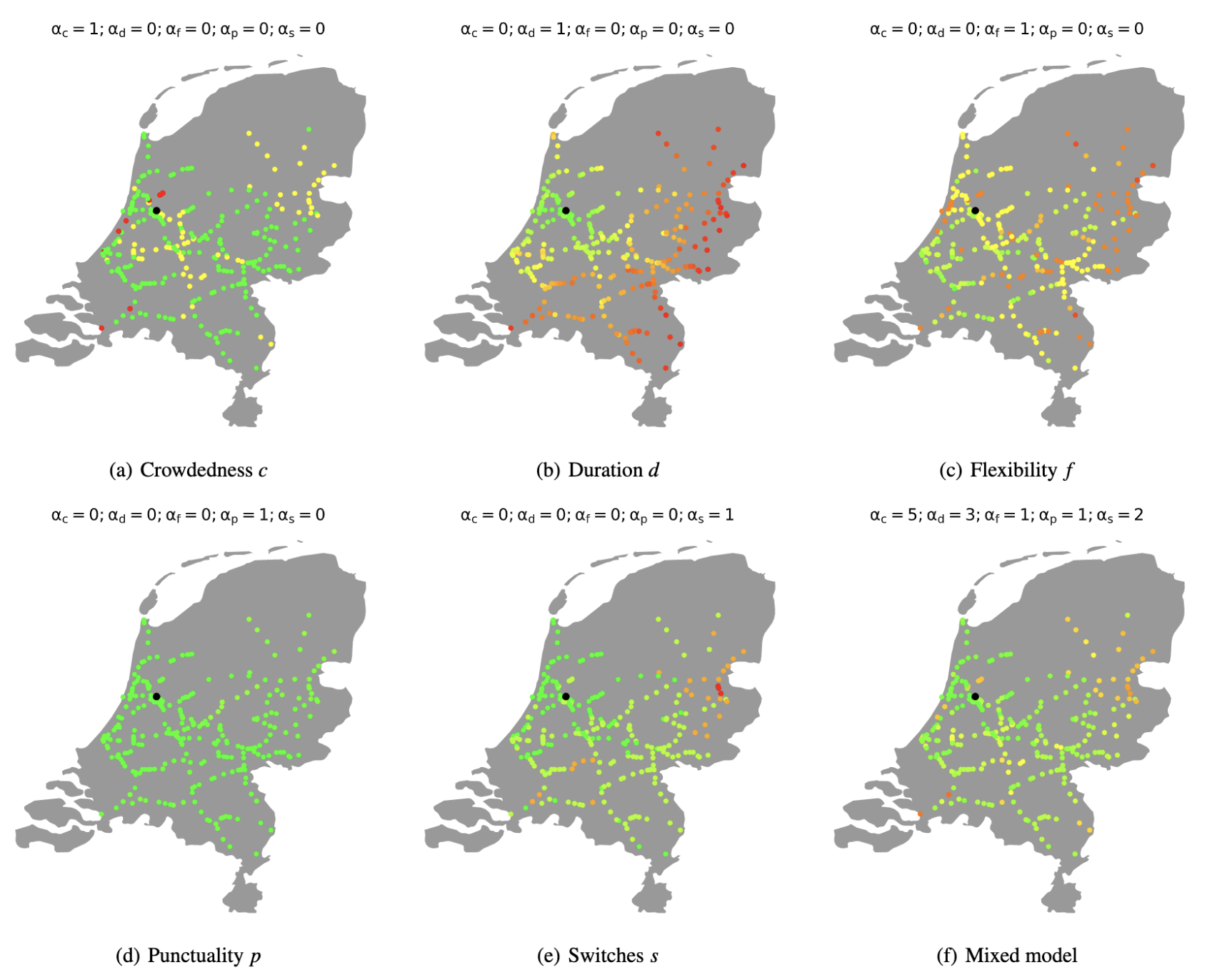Leon Overweel

# Modeling Train Commuter Stress for Dutch Cities

This is the project I did for the Social and Technological Networks course I took as part of my MSc Artificial Intelligence at the University of Edinbrugh. Using data from the Nederlandse Spoorwegen, I modeled which cities are best to commute from if you work near Amsterdam Central Station (ASD) but don't want to pay Amsterdam rent, based on the following factors:

• Crowdedness $$c$$: How busy the train is
• Duration $$d$$: How much time it takes to get to ASD
• Flexibility $$f$$: How many ways there are to get to ASD
• Punctuality $$p$$: Whether the train(s) will arrive on time
• Switches $$l$$: How many times the commuter has to switch trains

I combined these factors into a single stress measure $$S$$ as follows, where $$\alpha$$s are weights for each factor and $$O$$ is the set of possible options:

$$S = \min_{\forall o \in O} \left( \alpha_c c_o + \alpha_d d_o + \alpha_p (1 - p_o) + \alpha_s s_o \right) + \alpha_f (1 - f)$$

By scraping data from the Dutch national railway operator Nationale Spoorwegen (NS), I could then make the following plots of how “stressful” a commute to Amsterdam would be for each train station in the Netherlands, for different $$\alpha$$ settings.Stress maps with different settings for $$\alpha$$s; green indicates low stress and red indicates high stress. Amsterdam Central Station (ASD) is marked in black. The mixed model uses my preferences as $$\alpha$$ settings.

Using settings from the bottom-right mixed model, the best cities to commute from are mostly in the Randstad and the area north-west of Amsterdam. See Appendix A of the report for a list of the top-thirty stations according to this metric.